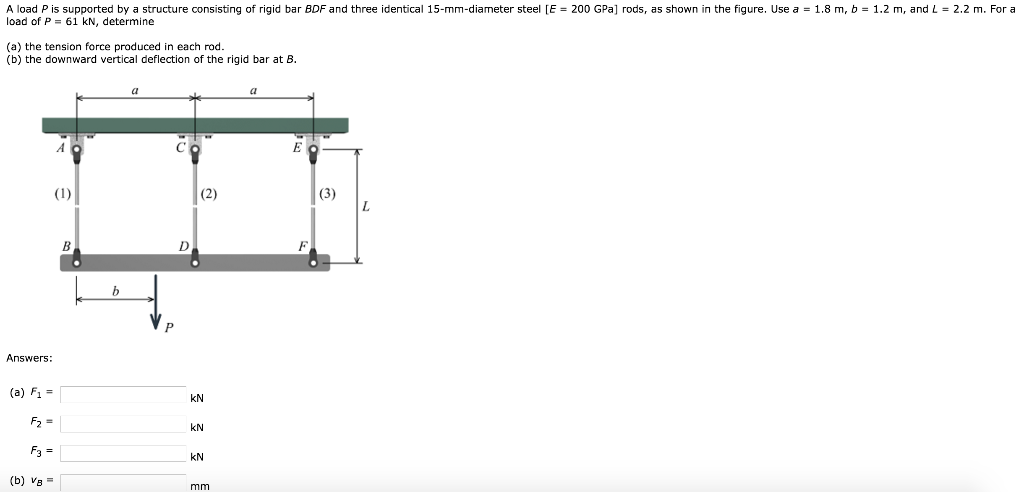# Question A load P is supported by a structure consisting of rigid bar BDF and three identical 15-mm-diameter steel [E = 200 GPa) rods, as shown in the figure. Use a = 1.8 m, b = 1.2 m, and L = 2.2 m. For a load of P = 61 kN, determine (a) the tension force produced in each rod. (b) the downward vertical deflection of the rigid bar at B. (0) Answers: (a) F1 = (b) Vg =